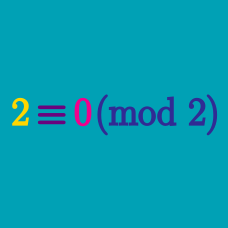Number Theory

# Modular Arithmetic Operations

#### Challenge Quizzes

March 1, 2016 is Tuesday. What day of the week is April 1, 2016?

6515 leaves a remainder of 4 upon division by 17.
3897 also leaves a remainder of 4 upon division by 17.

Then what is the remainder of 2618 upon division by 17?

What is equivalent to $703 - 564 \pmod{7}$?

What is $\underbrace{7+7+7+\ldots+7}_{\text{100 7's}}\ \bmod{6}?$

What is $(83 + 23) \bmod{4}$?

×